# Equation of Circle

Equation of Circle
Go back to  'Circles'

In this mini-lesson, we will learn about the equation of a circle by understanding the different ways of representing a circle and the ways to represent a circle on a graph. We will also discover interesting facts around the equation of a circle.

Joy is playing with a rope in a playground. He ties the rope to a point on the ground. He ties a stick to the other free end of the rope.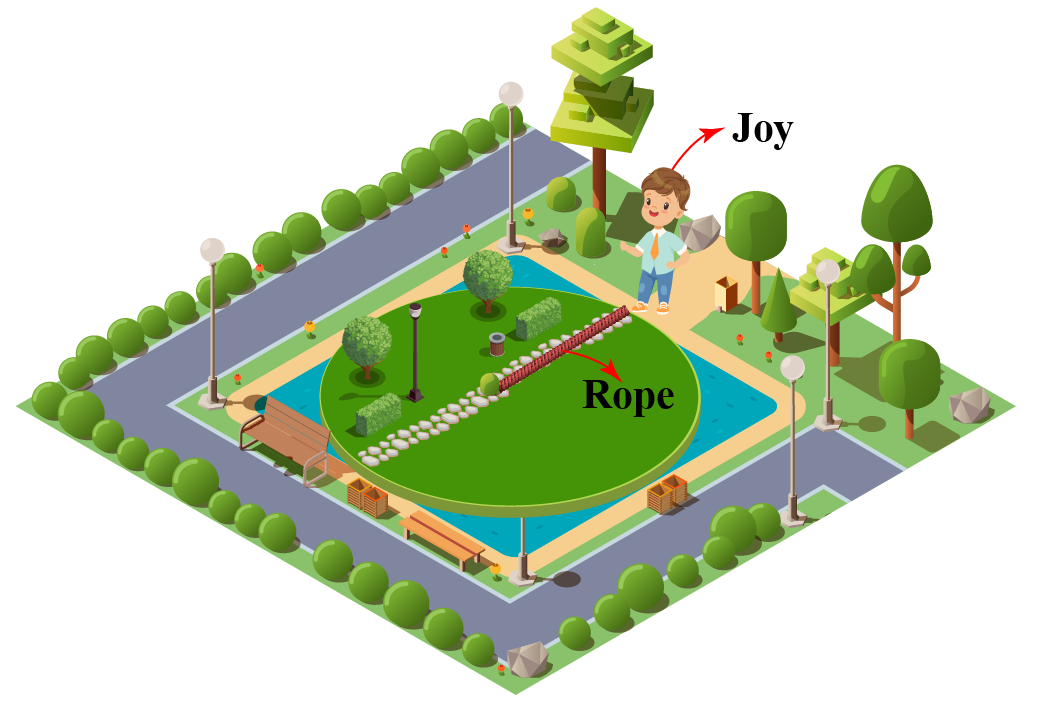He stretches the rope as much as he can and starts moving with the rope and stick, such that the stick marks the path. He realizes after some time that he has reached the same point from where he had started. He also realizes that the path traced by the stick, as he is moving at a fixed distance from a point, is nothing but a circle.

Have you ever come across the equation of a circle when the coordinates of the center and the radius are given?

Play with the simulation below and explore the different equations of a circle.

## Lesson Plan

 1 What Is a Circle? 2 Tips and Tricks 3 Important Notes on Equation of Circle 4 Solved Examples on Equation of Circle 5 Interactive Questions on Equation of Circle

## What Is a Circle?

A circle is a figure obtained by joining all the points in a plane such that every point is at an equal distance from the point at the center.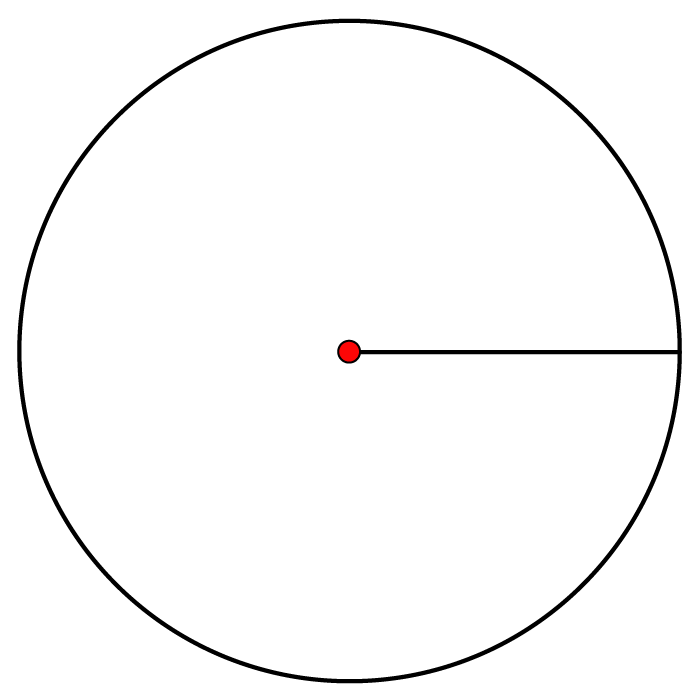Radius of a circle is the distance between the center and any point on the boundary of the circle.

## Circle on a Graph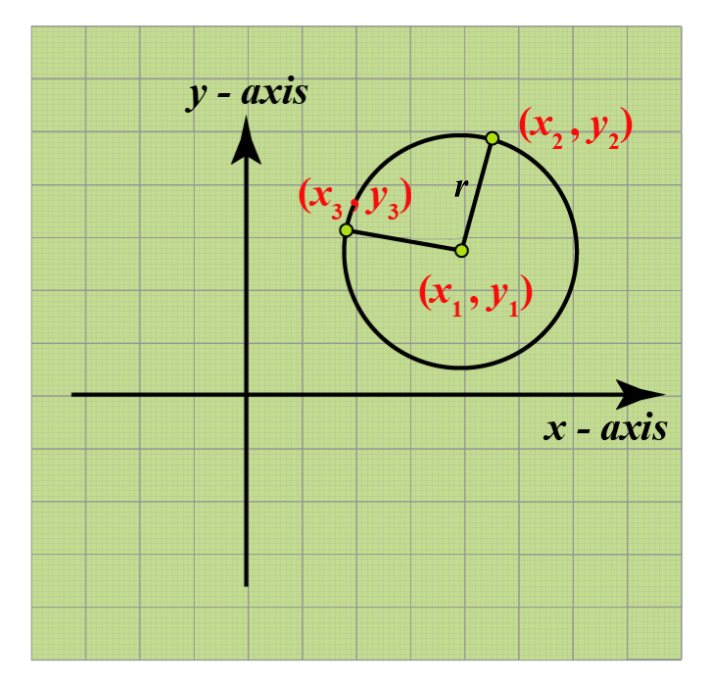In the figure above, we can see a circle on a graph.

The center of the circle is point $$(x_1, y_1)$$, and the points $$(x_2, y_2)$$ and $$(x_3, y_3)$$ are the two points on the circumference of the circle.

Radius $$(r)$$ of the circle is the distance between the center and any one of the two points.

$$r = \sqrt{(y_2 - y_1)^2 + (x_2 - x_1)^2}$$ or $$r = \sqrt{(y_3 - y_1)^2 + (x_3 - x_1)^2}$$

## What Is the Standard Form of Equation of a Circle?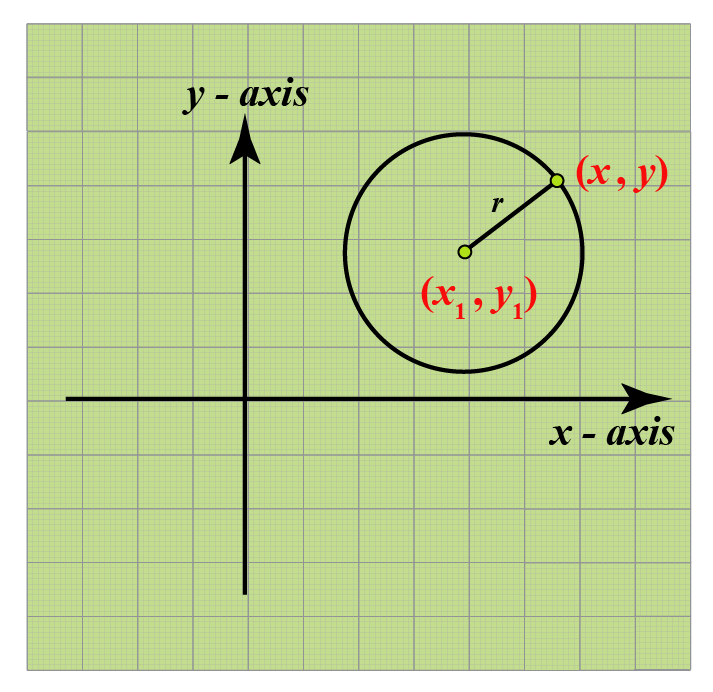$$(x_1, y_1)$$ is the center of the circle with radius $$r$$.

$$(x, y)$$ is an arbitrary point on the circumference of the circle.

The distance between this point and the center is equal to the radius of the circle.

Let's apply the distance formula between these points.

$$\sqrt{(x - x_1)^2 + (y - y_1)^2} = r$$

Squaring both sides, we get:

$$(x - x_1)^2 + (y - y_1)^2 = r^2$$

This is the standard form of the equation of a circle.

 Standard form $$(x - x_1)^2 + (y - y_1)^2 = r^2$$

Example

Write the equation of a circle with center $$(-1, 2)$$ and radius equal to 3 units in the standard form.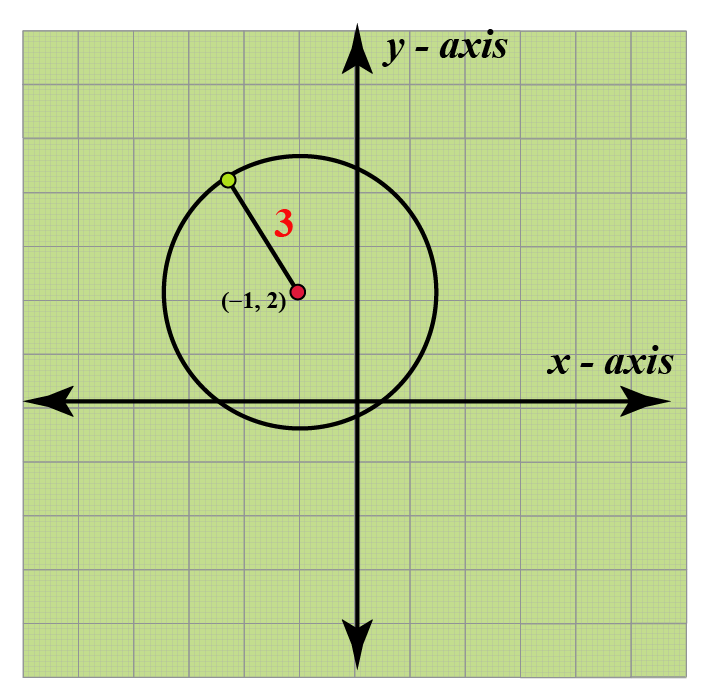Solution

Equation for a circle in standard form is given as:

$$(x - x_1)^2 + (y - y_1)^2 = r^2$$

where $$(x_1, y_1) = (-1, 2)$$ is the center of the circle and $$r = 3$$ is the radius of the circle.

Substituting the values in the equation

\begin{align} (x - (-1))^2 + (y - 2)^2 &= 3^2\0.2cm] (x + 1)^2 + (y - 2)^2 &= 9\\[0.2cm] \end{align}  $$(x + 1)^2 + (y - 2)^2 = 9$$ ### Center at Origin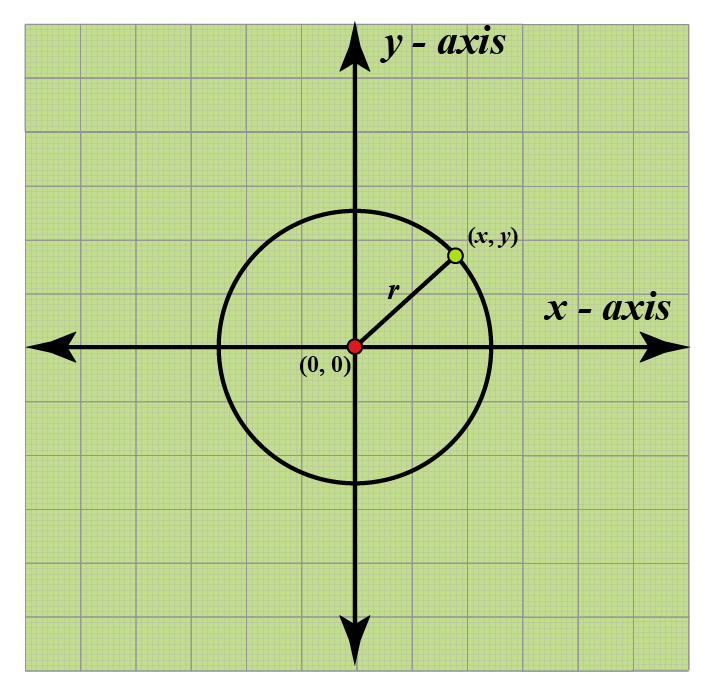In the above figure, $$(0, 0)$$ is the center of the circle with radius $$r$$. $$(x, y)$$ is an arbitrary point on the circumference of the circle. The distance between this point and the center is equal to the radius of the circle. Let's apply the distance formula between these points. $$\sqrt{(x - 0)^2 + (y - 0)^2} = r$$ Squaring both sides, we get: $$(x - 0)^2 + (y - 0)^2 = r^2$$ $$x^2 + y^2 = r^2$$  $$x^2 + y^2 = r^2$$ ### Center on X-axis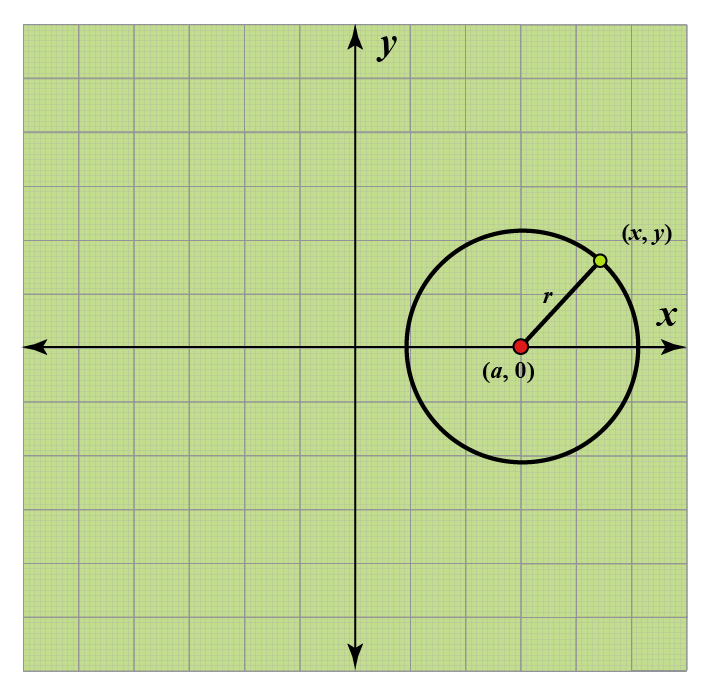$$(a, 0)$$ is the center of the circle with radius $$r$$. $$(x, y)$$ is an arbitrary point on the circumference of the circle. The distance between this point and the center is equal to the radius of the circle. Let's apply the distance formula between these points. $$\sqrt{(x - a)^2 + (y - 0)^2} = r$$ Squaring both sides, we get: $$(x - a)^2 + (y - 0)^2 = r^2$$ $$(x - a)^2 + (y)^2 = r^2$$  $$(x - a)^2 + y^2 = r^2$$ ### Center on Y-axis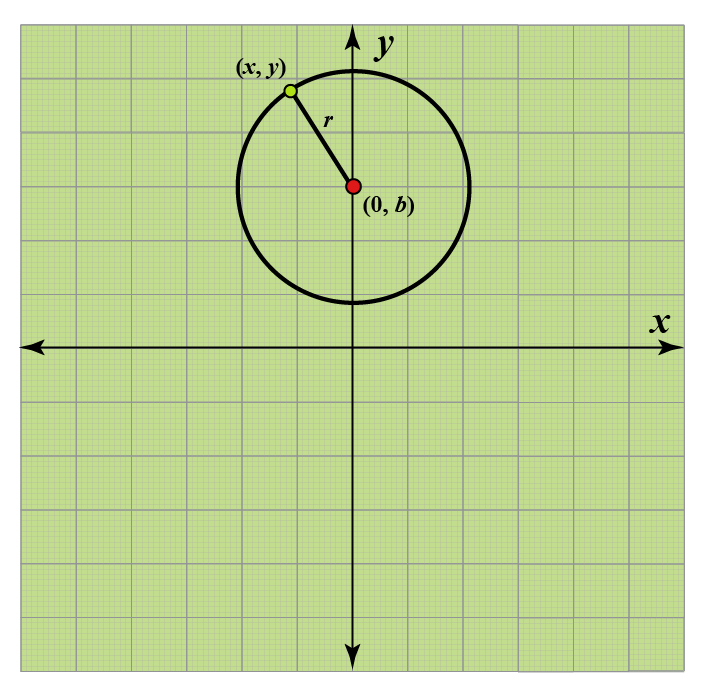$$(0, b)$$ is the center of the circle with radius $$r$$. $$(x, y)$$ is an arbitrary point on the circumference of the circle. The distance between this point and the center is equal to the radius of the circle. Let's apply the distance formula between these points. $$\sqrt{(x - 0)^2 + (y - b)^2} = r$$ Squaring both sides, we get: $$(x - 0)^2 + (y - b)^2 = r^2$$ $$(x)^2 + (y - b)^2 = r^2$$  $$x^2 + (y - b)^2 = r^2$$ ### Circle Touching X-axis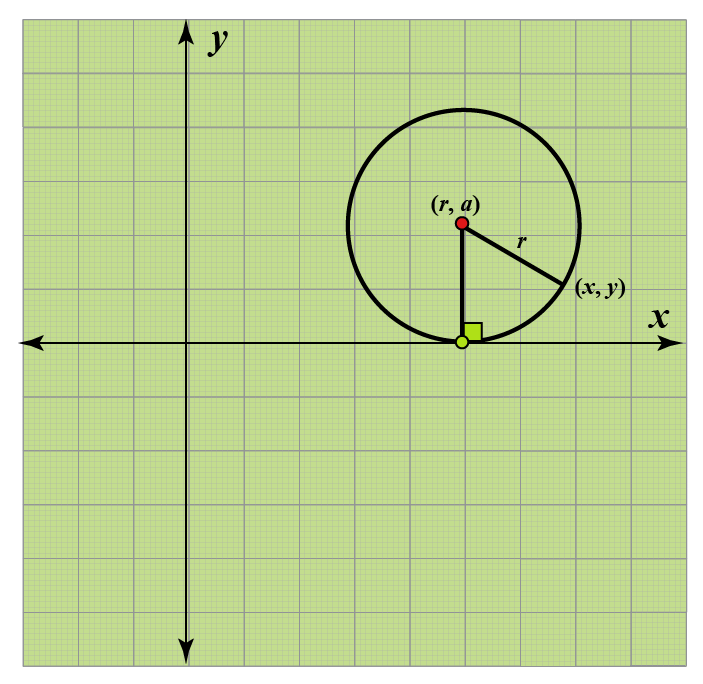$$(a, r)$$ is the center of the circle with radius $$r$$. If a circle touches the X-axis, then the y-coordinate of the center of the circle is equal to the radius $$r$$. $$(x, y)$$ is an arbitrary point on the circumference of the circle. The distance between this point and the center is equal to the radius of the circle. Let's apply the distance formula between these points. $$\sqrt{(x - a)^2 + (y - r)^2} = r$$ Squaring both sides, we get: $$(x - a)^2 + (y - r)^2 = r^2$$  $$(x - a)^2 + (y - r)^2 = r^2$$ ### Circle Touching Y-axis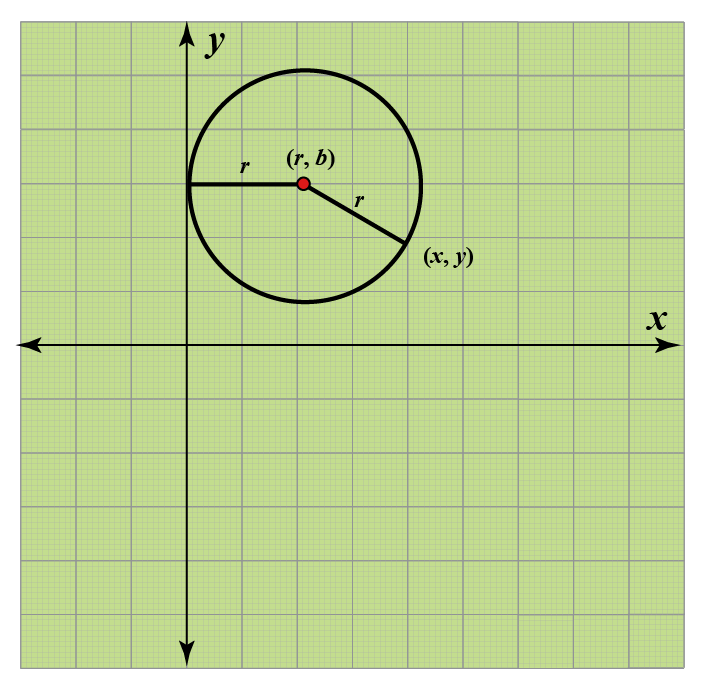$$(r, b)$$ is the center of the circle with radius $$r$$. If a circle touches the Y-axis, then the x-coordinate of the center of the circle is equal to the radius $$r$$. $$(x, y)$$ is an arbitrary point on the circumference of the circle. The distance between this point and the center is equal to the radius of the circle. Let's apply the distance formula between these points. $$\sqrt{(x - r)^2 + (y - b)^2} = r$$ Squaring both sides $$(x - r)^2 + (y - b)^2 = r^2$$  $$(x - r)^2 + (y - b)^2 = r^2$$ ### Circle Touching Both X-axis and Y-axis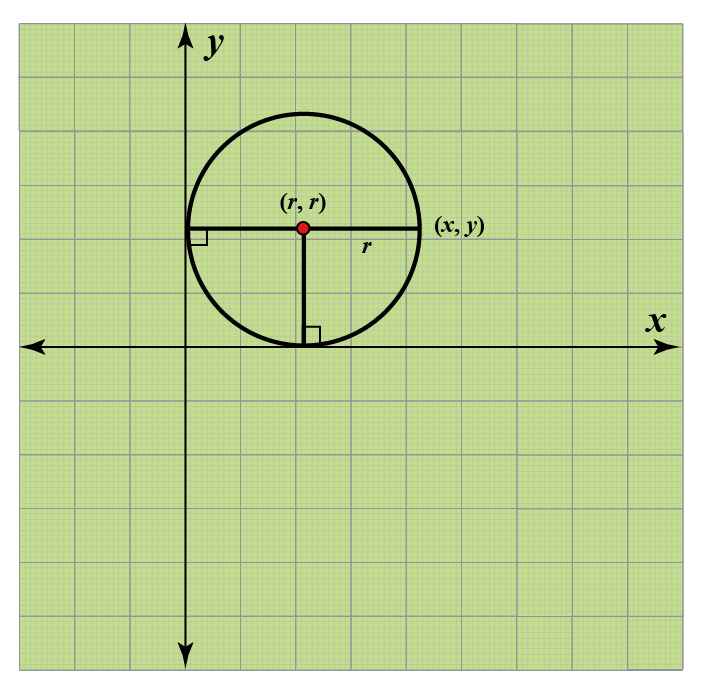$$(r, r)$$ is the center of the circle with radius $$r$$. If a circle touches both X-axis and Y-axis, then the coordinates of the center of the circle is equal to the radius $$(r, r)$$. $$(x, y)$$ is an arbitrary point on the circumference of the circle. The distance between this point and the center is equal to the radius of the circle. Let's apply the distance formula between these points. $$\sqrt{(x - r)^2 + (y - r)^2} = r$$ Squaring both sides $$(x - r)^2 + (y - r)^2 = r^2$$  $$(x - r)^2 + (y - r)^2 = r^2$$Tips and Tricks • If a circle touches both the axes, then consider the center of the circle to be $$(r, r)$$, where $$r$$ is the radius of the circle. Here, $$(r, r)$$ can be positive as well as negative. For example, the radius of the circle is 3 and it is touching both the axes, then the coordinates of the center can be $$(3, 3)$$, $$(3, -3)$$, $$(-3, 3)$$, or $$(-3, -3)$$. • A tangent touches a circle at one point. Hence, the point of contact of the tangent satisfies the equation of the circle as well as the equation of the tangent. ## Conversion From Standard Form to the General Form Standard form of the equation of a circle is: $$(x - x_1)^2 + (y - y_1)^2 = r^2$$ Let's expand the above equation using the identities. $$x^2 +{x_1}^2 -2xx_1 + y^2 +{y_1}^2 -2yy_1 = r^2$$ $$x^2 + y^2 - 2xx_1 - 2yy_1 + {x_1}^2 + {y_1}^2 = r^2$$ $$x^2 + y^2 - 2xx_1 - 2yy_1 + {x_1}^2 + {y_1}^2 -r^2 = 0$$ Replace $$-2x_1$$ with $$A$$, $$-2y_1$$ with $$B$$, $${x_1}^2 + {y_1}^2 -r^2$$ with $$C$$ $$x^2 + y^2 + Ax + By + C = 0$$ General form of equation of circle is:  General form $$x^2 + y^2 + Ax + By + C = 0$$ Where A, B, C are constants ## Solved Examples  Example 1 Gerry's friend gave him an equation of a circle $$x^2 + y^2 +6x + 8y + 9 = 0$$ and asked him to find the coordinates of the center and the radius of the circle. Help him solve the problem. Solution Given the equation of the circle $$x^2 + y^2 +6x + 8y + 9 = 0$$ The general form of the equation of the circle with center $$(x_1, y_1)$$ and radius $$r$$ is $$x^2 + y^2 + Ax + By + C = 0$$ where $$A = -2x_1$$ $$B = -2y_1$$ $$C = {x_1}^2 + {y_1}^2 -r^2$$ From the equation of the circle $$x^2 + y^2 +6x + 8y + 9 = 0$$ \[\begin{align} A &= 6 \\[0.2cm] -2x_1 &= 6 \\[0.2cm] x_1 &= -3 \\[0.2cm] B &= 8 \\[0.2cm] -2y_1 &= 8 \\[0.2cm] y_1 &= -4 \\[0.2cm] C &= 9 \\[0.2cm] {x_1}^2 + {y_1}^2 -r^2 &= 9 \\[0.2cm] {-3}^2 + {-4}^2 -r^2 &= 9 \\[0.2cm] 9 + 16 -r^2 &= 9 \\[0.2cm] r^2 &= 16 \\[0.2cm] r &= 4 \\[0.2cm] \end{align}

 $$\therefore$$ Center of the circle is (-3, -4) and radius is 4 units
 Example 2

Find the point of intersection of the circle $$x^2 + y^2 -7x -8y +12 = 0$$ and the axes.

Solution

If the circle crosses the X-axis, the value of the y-coordinate is zero

Substitute y = 0 in the equation of circle
\begin{align} x^2 + y^2 -7x -8y +12 &= 0 \\[0.2cm] x^2 + 0^2 -7x -8 \times 0 +12 &= 0 \\[0.2cm] x^2 -7x +12 &= 0 \\[0.2cm] (x -3)(x-4) &= 0 \\[0.2cm] x &= 3 \\[0.2cm] x &= 4 \\[0.2cm] \end{align}
Hence, the circle crosses the x-axis at $$(3, 0), (4, 0)$$.

If the circle crosses the Y-axis, the value of the x-coordinate is zero.

Substitute x = 0 in the equation of circle
\begin{align} x^2 + y^2 -7x -8y +12 &= 0 \\[0.2cm] 0^2 + y^2 -7 \times 0 - 8y +12 &= 0 \\[0.2cm] y^2 - 8y +12 &= 0 \\[0.2cm] (y - 6)(y - 2) &= 0 \\[0.2cm] y &= 6 \\[0.2cm] y &= 2 \\[0.2cm] \end{align}
Hence, the circle crosses the y-axis at $$(0, 2), (0, 6)$$.

 $$\therefore$$ The circle crosses the axes at $$(3, 0), (4, 0), (0, 2), (0, 6)$$

More Important Topics
Numbers
Algebra
Geometry
Measurement
Money
Data
Trigonometry
Calculus